# 22 K-Means Clustering¶

Here is some two-dimensional data samples.

In :
import numpy as np
import matplotlib.pyplot as plt
import pandas

In :
data = np.array([[8,7],[7,6.6],[9.2,8.3],[6.8,9.2], [1.2,3.2],[4.8,2.3],[3.4,3.2],[3.2,5.6],[1,4],[2,2.2]])

In :
plt.scatter(data[:, 0], data[:, 1]);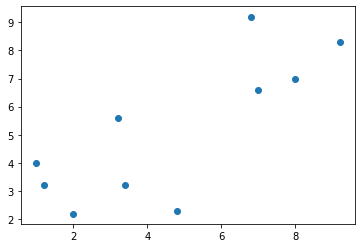First, let's try to find two clusters, so $k=2$. We must initialize the two means of the two clusters. Let's just pick two samples at random.

In :
np.random.choice(range(data.shape), 2, replace=False) # data.shape is number of rows, or samples

Out:
array([2, 6])
In :
np.random.choice(range(data.shape), 2, replace=False)

Out:
array([5, 2])
In :
centersIndex = np.random.choice(range(data.shape),2 , replace=False)
centersIndex

Out:
array([0, 2])
In :
centers = data[centersIndex, :]
centers

Out:
array([[8. , 7. ],
[9.2, 8.3]])

Now we must find all samples that are closest to the first center, and those that are closest to the second sample.

In :
a = np.array([1, 2, 3])
b = np.array([10, 20, 30])
a, b

Out:
(array([1, 2, 3]), array([10, 20, 30]))
In :
a - b

Out:
array([ -9, -18, -27])

But what if we want to subtract every element of a with every element of b?

In :
np.resize(a, (3, 3))

Out:
array([[1, 2, 3],
[1, 2, 3],
[1, 2, 3]])
In :
np.resize(b, (3, 3))

Out:
array([[10, 20, 30],
[10, 20, 30],
[10, 20, 30]])
In :
np.resize(a,(3, 3)).T

Out:
array([[1, 1, 1],
[2, 2, 2],
[3, 3, 3]])
In :
np.resize(a, (3, 3)).T - np.resize(b, (3, 3))

Out:
array([[ -9, -19, -29],
[ -8, -18, -28],
[ -7, -17, -27]])

However, we can ask numpy to do this duplication for us if we reshape a to be a column vector and leave b as a row vector.

$$\begin{pmatrix} 1\\ 2\\ 3 \end{pmatrix} - \begin{pmatrix} 10 & 20 & 30 \end{pmatrix} \;\; = \;\; \begin{pmatrix} 1 & 1 & 1\\ 2 & 2 & 2\\ 3 & 3 & 3 \end{pmatrix} - \begin{pmatrix} 10 & 20 & 30\\ 10 & 20 & 30\\ 10 & 20 & 30 \end{pmatrix}$$
In :
a = a.reshape((-1, 1))
a

Out:
array([,
,
])
In :
a - b

Out:
array([[ -9, -19, -29],
[ -8, -18, -28],
[ -7, -17, -27]])
In :
a = np.array([1, 2, 3])
b = np.array([[10, 20, 30], [40, 50, 60]])
print(a)
print(b)

[1 2 3]
[[10 20 30]
[40 50 60]]

In :
b - a

Out:
array([[ 9, 18, 27],
[39, 48, 57]])

The single row vector a is duplicated for as many rows as there are in b! We can use this to calculate the squared distance between a center and every sample.

In :
centers[0, :]  # zero-th row of centers

Out:
array([8., 7.])
In :
np.sum((centers[0, :] - data) ** 2, axis=1)

Out:
array([ 0.  ,  1.16,  3.13,  6.28, 60.68, 32.33, 35.6 , 25.  , 58.  ,
59.04])

Now we can test, for each sample, if the sample is closest to the first center.

In :
np.sum((centers[1, :] - data) ** 2, axis=1) > np.sum((centers[0, :] - data) ** 2, axis=1)

Out:
array([ True,  True, False,  True,  True,  True,  True,  True,  True,
True])
In :
centers

Out:
array([[8. , 7. ],
[9.2, 8.3]])

We want something a little more general, so it works for as many centers as we have rows in centers.

In :
centers[:, np.newaxis, :].shape, data.shape

Out:
((2, 1, 2), (10, 2))
In :
(centers[:, np.newaxis, :] - data).shape

Out:
(2, 10, 2)
In :
np.sum((centers[:, np.newaxis, :] - data) ** 2, axis=2).shape

Out:
(2, 10)
In :
np.argmin(np.sum((centers[:, np.newaxis, :] - data) ** 2, axis=2), axis=0)

Out:
array([0, 0, 1, 0, 0, 0, 0, 0, 0, 0])

So we can now assign to each sample which center it is closest to. All samples closest to a particular center forms a cluster.

In :
cluster = np.argmin(np.sum((centers[:,np.newaxis,:] - data)**2, axis=2), axis=0)
cluster

Out:
array([0, 0, 1, 0, 0, 0, 0, 0, 0, 0])

Once we have collected all of the samples in one cluster, we can calculate a new center for that cluster as the mean of all of the samples in that cluster.

In :
data[cluster == 0, :].mean(axis=0)

Out:
array([4.15555556, 4.81111111])
In :
data[cluster == 1, :].mean(axis=0)

Out:
array([9.2, 8.3])
In :
k = 2
for i in range(k):
centers[i, :] = data[cluster == i, :].mean(axis=0)

In :
centers

Out:
array([[4.15555556, 4.81111111],
[9.2       , 8.3       ]])
In :
def kmeans(data, k = 2, n_iterations = 5):

# Initial centers
centers = data[np.random.choice(range(data.shape), k, replace=False), :]

# Repeat n times
for iteration in range(n_iterations):

# Which center is each sample closest to?
closest = np.argmin(np.sum((centers[:, np.newaxis, :] - data) ** 2, axis=2), axis=0)

# Update cluster centers
for i in range(k):
centers[i, :] = data[closest == i, :].mean(axis=0)

return centers, closest

In :
kmeans(data, k=2, n_iterations=5)

Out:
(array([[7.75      , 7.775     ],
[2.6       , 3.41666667]]),
array([0, 0, 0, 0, 1, 1, 1, 1, 1, 1]))
In :
kmeans(data, k=2, n_iterations=5)

Out:
(array([[7.75      , 7.775     ],
[2.6       , 3.41666667]]),
array([0, 0, 0, 0, 1, 1, 1, 1, 1, 1]))
In :
centers, closest = kmeans(data, k=2, n_iterations=2)
plt.scatter(data[:, 0], data[:, 1], s=80, c=closest, alpha=0.5);
plt.scatter(centers[:, 0], centers[:, 1], s=80, c='green', alpha=0.5);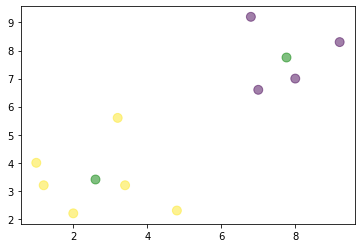Why does the second time we call kmeans produce a different answer?

Let's define $J$ as the performance measure being minimized by k-means. $$J = \sum_{n=1}^N \sum_{k=1}^K r_{nk} ||\mathbf{x}_n - \mathbf{\mu}_k||^2$$ where $N$ is the number of samples, $K$ is the number of cluster centers, $\mathbf{x}_n$ is the $n^{th}$ sample and $\mathbf{\mu}_k$ is the $k^{th}$ center, each being an element of $\mathbf{R}^p$ where $p$ is the dimensionality of the data, and $r_{nk}$ is 1 if cluster $k$ is closest to sample $n$, 0 otherwise.

The sums can be computed using python for loops, but for loops are much slower than matrix operations in python, as the following cells show.

In :
a = np.linspace(0, 10, 30).reshape(3, 10)
a

Out:
array([[ 0.        ,  0.34482759,  0.68965517,  1.03448276,  1.37931034,
1.72413793,  2.06896552,  2.4137931 ,  2.75862069,  3.10344828],
[ 3.44827586,  3.79310345,  4.13793103,  4.48275862,  4.82758621,
5.17241379,  5.51724138,  5.86206897,  6.20689655,  6.55172414],
[ 6.89655172,  7.24137931,  7.5862069 ,  7.93103448,  8.27586207,
8.62068966,  8.96551724,  9.31034483,  9.65517241, 10.        ]])
In :
b = np.arange(30).reshape(3, 10)
b

Out:
array([[ 0,  1,  2,  3,  4,  5,  6,  7,  8,  9],
[10, 11, 12, 13, 14, 15, 16, 17, 18, 19],
[20, 21, 22, 23, 24, 25, 26, 27, 28, 29]])
In :
result = np.zeros((3, 10))
for i in range(3):
for j in range(10):
result[i, j] = a[i, j] + b[i, j]
result

Out:
array([[ 0.        ,  1.34482759,  2.68965517,  4.03448276,  5.37931034,
6.72413793,  8.06896552,  9.4137931 , 10.75862069, 12.10344828],
[13.44827586, 14.79310345, 16.13793103, 17.48275862, 18.82758621,
20.17241379, 21.51724138, 22.86206897, 24.20689655, 25.55172414],
[26.89655172, 28.24137931, 29.5862069 , 30.93103448, 32.27586207,
33.62068966, 34.96551724, 36.31034483, 37.65517241, 39.        ]])
In :
a.shape, a[:, np.newaxis].shape

Out:
((3, 10), (3, 1, 10))

Now, back to our problem. How can we use matrix operations to calculate the squared distance between two centers and, say, five data samples? Let's say both are two-dimensional.

In :
centers = np.array([[1, 2], [5, 4]])
centers

Out:
array([[1, 2],
[5, 4]])
In :
data = np.array([[3, 2], [4, 6], [7, 3], [4, 6], [1, 8]])
data

Out:
array([[3, 2],
[4, 6],
[7, 3],
[4, 6],
[1, 8]])

This will be a little weird, and hard to understand, but by adding an empty dimension to the centers array, numpy broadcasting does all the work for us.

In :
centers[:, np.newaxis, :]

Out:
array([[[1, 2]],

[[5, 4]]])
In :
centers[:, np.newaxis, :].shape

Out:
(2, 1, 2)
In :
data.shape

Out:
(5, 2)
In :
diffsq = (centers[:, np.newaxis, :] - data) ** 2
diffsq

Out:
array([[[ 4,  0],
[ 9, 16],
[36,  1],
[ 9, 16],
[ 0, 36]],

[[ 4,  4],
[ 1,  4],
[ 4,  1],
[ 1,  4],
[16, 16]]])
In :
diffsq.shape

Out:
(2, 5, 2)
In :
np.sum(diffsq, axis=2)

Out:
array([[ 4, 25, 37, 25, 36],
[ 8,  5,  5,  5, 32]])

Now we have a 2 x 5 array with the first row containing the squared distance from the first center to each of the five data samples, and the second row containing the squared distances from the second center to each of the five data samples.

Now we just have to find the smallest distance in each column and sum them up.

In :
np.min(np.sum(diffsq, axis=2), axis=0)

Out:
array([ 4,  5,  5,  5, 32])
In :
np.sum(np.min(np.sum(diffsq, axis=2), axis=0))

Out:
51

Let's define a function named calcJ to do this calculation.

In :
def calcJ(data, centers, not_empty=None):

if  not_empty is None:
not_empty = [True] * centers.shape
diffsq = (centers[np.array(not_empty), np.newaxis, :] - data) ** 2
return np.sum(np.min(np.sum(diffsq, axis=2), axis=0))

In :
calcJ(data, centers)

Out:
51
In :
def kmeans(data, k = 2, n_iterations = 5):

# Initialize centers and list J to track performance metric
centers = data[np.random.choice(range(data.shape), k, replace=False), :]
J = []
# Calculate J and append to list J
J = [calcJ(data, centers, not_empty=None)]

# Repeat n times
for iteration in range(n_iterations):

# Which center is each sample closest to?
sqdistances = np.sum((centers[:, np.newaxis, :] - data) ** 2, axis=2)
closest = np.argmin(sqdistances, axis=0)
if iteration == 0:
last_closest = closest.copy()
else:
if np.all(closest == last_closest):
break

# Update cluster centers
not_empty = [True] * k
for i in range(k):
if np.sum(closest == i) == 0:
not_empty[i] = False
else:
centers[i, :] = data[closest==i, :].mean(axis=0)

# Calculate J one final time and return results
J.append(calcJ(data, centers, not_empty))

return centers, J, closest, not_empty

In :
centers, J, closest, not_empty = kmeans(data, k=2, n_iterations=5)

In :
not_empty

Out:
[True, True]
In :
J

Out:
[35, 23]
In :
plt.plot(J);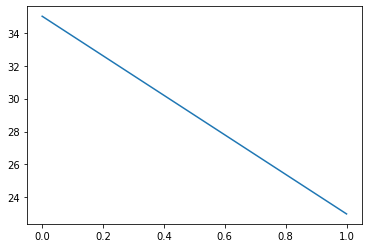In :
centers, J, closest, not_empty = kmeans(data, k=2, n_iterations=10)
plt.plot(J);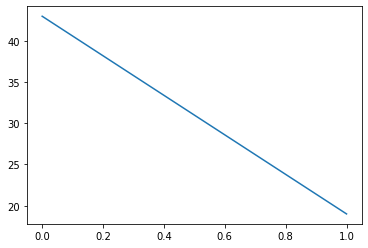In :
data.shape

Out:
(5, 2)
In :
centers, J, closest, not_empty = kmeans(data, k=5, n_iterations=10)
plt.plot(J);
not_empty

Out:
[True, True, True, True, False]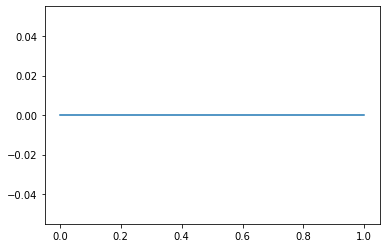## Old Faithful Data¶

Let's represent clusters as a list of sample matrices, each matrix containing samples from one cluster. Initially, all samples are in their own clusters. Let's use the Old Faithful data to develop our implementation. Download it from here.

In :
!head oldfaithful.csv

3.600      79
1.800      54
3.333      74
2.283      62
4.533      85
2.883      55
4.700      88
3.600      85
1.950      51
4.350      85

In :
data = np.loadtxt('oldfaithful.csv')

In :
data.shape

Out:
(272, 2)
In :
plt.scatter(data[:,0],data[:,1]);
plt.xlabel('Duration')
plt.ylabel('Interval');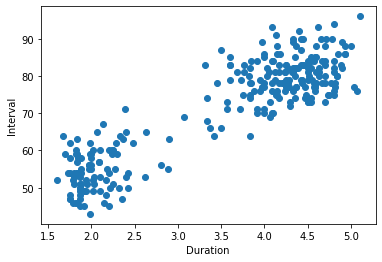In :
k = 10
centers, J, closest, not_empty = kmeans(data, k=k, n_iterations=20)

print(f'k={k} n_not_empty={sum(not_empty)}')
plt.plot(J);

k=10 n_not_empty=10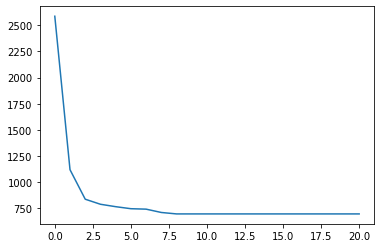In :
plt.scatter(data[:,0],data[:,1]);
plt.xlabel('Duration')
plt.ylabel('Interval')

plt.plot(centers[:, 0], centers[:, 1], 'ro', ms=10)

Out:
[<matplotlib.lines.Line2D at 0x7f92826e1c90>]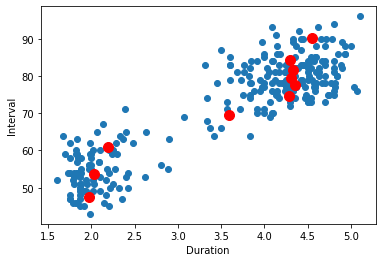In :
k = 3
centers, J, closest, not_empty = kmeans(data, k=k, n_iterations=20)

print(f'k={k} n_not_empty={sum(not_empty)}')
plt.plot(J);

plt.figure()

plt.scatter(data[:,0],data[:,1]);
plt.xlabel('Duration')
plt.ylabel('Interval')

plt.plot(centers[:, 0], centers[:, 1], 'ro', ms=10);

k=3 n_not_empty=3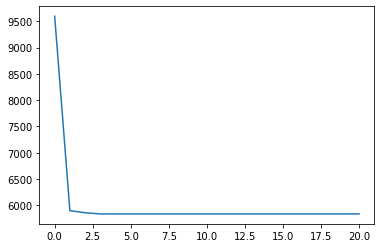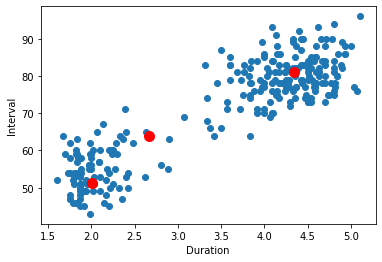In :
k = 2
centers, J, closest, not_empty = kmeans(data, k=k, n_iterations=20)

print(f'k={k} n_not_empty={sum(not_empty)}')
plt.plot(J);

plt.figure()

plt.scatter(data[:,0],data[:,1]);
plt.xlabel('Duration')
plt.ylabel('Interval')

plt.plot(centers[:, 0], centers[:, 1], 'ro', ms=10);

k=2 n_not_empty=2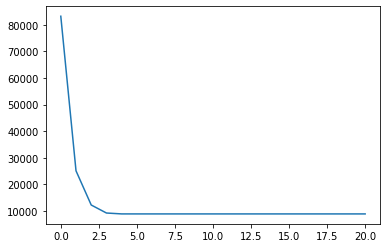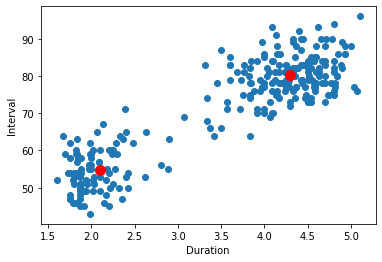## MNIST Data¶

In :
import gzip
import pickle

with gzip.open('mnist.pkl.gz', 'rb') as f:
train_set, valid_set, test_set = pickle.load(f, encoding='latin1')

X = train_set
T = train_set.reshape((-1,1))

Xtest = test_set
Ttest = test_set.reshape((-1,1))

X.shape, T.shape, Xtest.shape, Ttest.shape

Out:
((50000, 784), (50000, 1), (10000, 784), (10000, 1))
In :
k = 10
centers, J, closest, not_empty = kmeans(X, k=k, n_iterations=20)
print(f'k={k} n_not_empty={sum(not_empty)}')

k=10 n_not_empty=10

In :
plt.plot(J);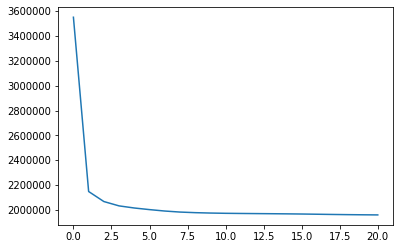In :
centers.shape

Out:
(10, 784)
In :
for i in range(10):
plt.subplot(2, 5 ,i + 1)
plt.imshow(-centers[i, :].reshape((28, 28)), cmap='gray')
plt.axis('off')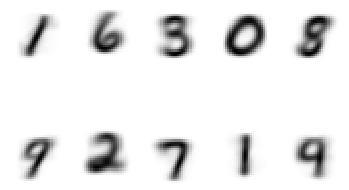In [ ]:
k = 10
centers, J, closest, not_empty = kmeans(X, k=k, n_iterations=20)
print(f'k={k} n_not_empty={sum(not_empty)}')
plt.plot(J)
plt.figure()
for i in range(k):
plt.subplot(2, 5, i + 1)
plt.imshow(-centers[i, :].reshape((28, 28)), cmap='gray')
plt.axis('off')

In :
k = 20
centers, J, closest, not_empty = kmeans(X, k=k, n_iterations=20)
print(f'k={k} n_not_empty={sum(not_empty)}')
plt.plot(J)
plt.figure()
for i in range(k):
plt.subplot(4, 5, i + 1)
plt.imshow(-centers[i, :].reshape((28, 28)), cmap='gray')
plt.axis('off')

k=20 n_not_empty=20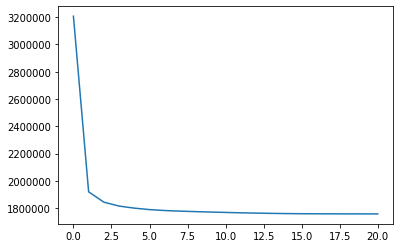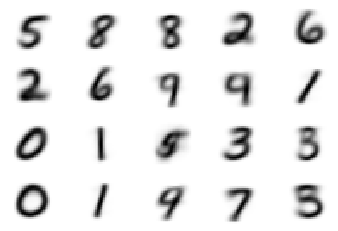Following code adapted from this site.

Can any of you figure out how to make the images of the centers appear in the first column??

In :
import io
import base64
from IPython.display import HTML

def create_figure(i):
fig, ax = plt.subplots(figsize=(4, 4))
ax.axis('off')
ax.imshow(-centers[i].reshape(28, 28), cmap='gray')
file = io.BytesIO()
fig.savefig(file, format='png')
file.seek(0)
png = base64.b64encode(file.getvalue())
imgstr = f'<img src="data:image/png;base64,{png}" />'
return imgstr

def mapping(i):
return create_figure(i)

k = centers.shape
df = pandas.DataFrame({'digit': [None] * k,
'0': np.zeros(k),
'1': np.zeros(k),
'2': np.zeros(k),
'3': np.zeros(k),
'4': np.zeros(k),
'5': np.zeros(k),
'6': np.zeros(k),
'7': np.zeros(k),
'8': np.zeros(k),
'9': np.zeros(k)})

for center_i, center in enumerate(centers):
df.iloc[0, 0] = center_i
uniques, counts = np.unique(T[closest == center_i], return_counts=True)
df.iloc[center_i, 1 + uniques] = 100 * counts / sum(counts)

pandas.set_option('display.max_colwidth', None, 'precision', 2)
HTML(df.to_html(escape=False, formatters=dict(digit=mapping)))

Out:
digit 0 1 2 3 4 5 6 7 8 9
03.67 0.00 0.06 1.78 0.12 87.27 2.07 0.00 4.38 0.65
1 None 1.26 0.26 3.92 5.71 0.26 1.05 0.17 1.35 84.58 1.44
2 None 0.67 0.45 5.88 22.27 0.18 2.36 0.36 0.94 65.35 1.56
3 None 0.82 0.00 92.06 3.51 1.03 0.05 0.26 0.62 0.82 0.82
4 None 3.76 0.05 1.33 0.38 2.24 0.81 90.48 0.14 0.57 0.24
5 None 0.19 0.82 95.44 1.68 0.05 0.14 0.67 0.53 0.48 0.00
6 None 5.44 0.14 1.54 0.67 2.42 1.76 87.26 0.00 0.67 0.11
7 None 0.12 0.14 0.47 1.50 24.07 1.10 0.05 33.12 0.56 38.86
8 None 0.18 0.03 1.44 0.88 47.03 2.50 0.47 13.13 1.06 33.28
9 None 0.00 84.66 3.85 0.70 1.52 0.64 0.70 4.61 2.57 0.76
10 None 92.15 0.00 1.52 0.62 0.19 1.57 2.81 0.29 0.48 0.38
11 None 0.00 83.07 2.04 3.29 1.33 0.98 0.82 3.25 2.55 2.66
12 None 3.99 0.77 10.63 3.77 14.31 35.86 8.13 5.41 8.75 8.38
13 None 0.65 0.05 3.92 83.12 0.00 5.99 0.16 0.00 5.23 0.87
14 None 9.19 0.63 4.60 50.63 0.00 23.41 1.96 0.43 7.93 1.22
15 None 95.90 0.00 0.48 0.31 0.09 0.74 1.09 0.26 0.57 0.57
16 None 0.00 84.92 2.78 0.88 1.01 0.46 1.31 5.18 2.40 1.05
17 None 0.10 0.00 0.07 0.16 49.85 0.42 0.10 2.25 2.38 44.66
18 None 0.00 0.10 1.58 0.69 0.16 0.13 0.00 88.56 0.36 8.40
19 None 2.09 0.00 0.84 46.64 0.00 32.26 0.54 0.08 16.26 1.30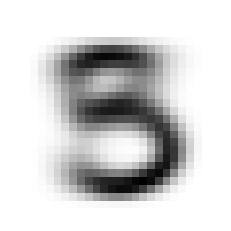In [ ]: